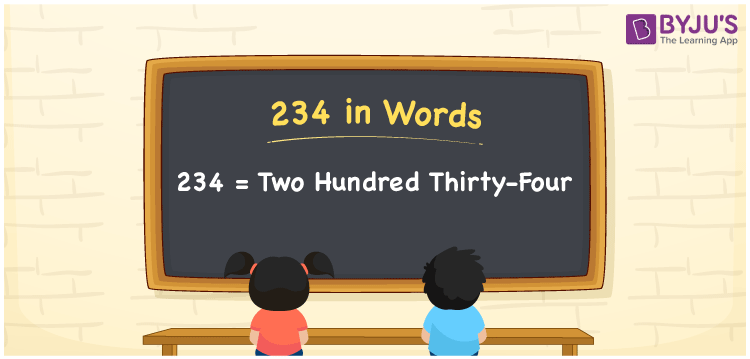# 234 in words

234 in words is written as Two Hundred and Thirty Four. 234 represents the count or value. The article on Counting Numbers can give you an idea about count or counting. The number 234 is a 3 digit number that is used in expressions related to money, days, distance, length, weight and so on. Let us consider an example for 234. “The shopkeeper cheated me by giving only Two Hundred and Thirty Four grams of rice instead of quarter kg”.

 234 in words Two Hundred and Thirty Four Two Hundred and Thirty Four in Numbers 234

## 234 in English Words## How to Write 234 in Words?

We can convert 234 to words using a place value chart. The number 234 has 3 digits, so let’s make a chart that shows the place value up to 3 digits.

 Hundreds Tens Ones 2 3 4

Thus, we can write the expanded form as:

2 × Hundred + 3 × Ten + 4 × One

= 2 × 100 + 3 × 10 + 4 × 1

= 234

= Two Hundred and Thirty Four.

234 is the natural number that is succeeded by 233 and preceded by 235.

234 in words – Two Hundred and Thirty Four.

Is 234 an odd number? – No.

Is 234 an even number? – Yes.

Is 234 a perfect square number? – No.

Is 234 a perfect cube number? – No.

Is 234 a prime number? – No.

Is 234 a composite number? – Yes.

## Solved Example

1. Write the number 234 in expanded form

Solution: 2 × 100 + 3 × 10 + 4 × 1

We can write 234 = 200 + 30 + 4

= 2 × 100 + 3 × 10 + 4 × 1.

## Frequently Asked Questions on 234 in words

Q1

### How to write the number 234 in words?

234 in words is written as Two Hundred and Thirty Four.
Q2

### Is 234 divisible by 3?

Yes. 234 is divisible by 3.
Q3

### Is 234 a perfect square number?

No. 234 is not a perfect square number.